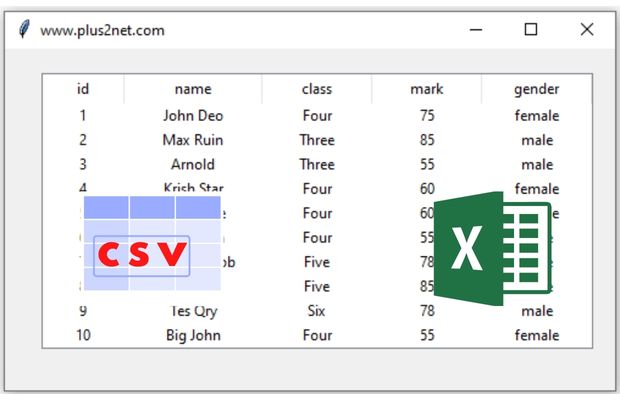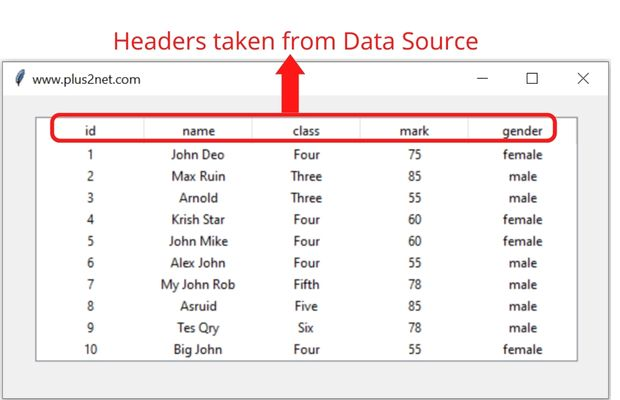# Dynamic Columns & headers of Treeview## Number of columns to display

When we collect data from the source ( database table or Excel or CSV file ) we are not sure how many columns will be available. Here are some SQL which collects different number of columns from the table
``````SELECT id,name FROM student
SELECT id,name,class FROM student
SELECT * FROM student``````
First and second query will collect two and three columns respectively from our student table. The third query will return all the columns of the student table.

This way we will not have any fixed number of columns and based on the source or data, we have to create the columns.

For our easy understanding let us create one list and use the elements as column headers. We can replace our list with data from Database table.
``l1=['id','Name','Class','Mark','Gender']``
Using this list we will add our columns and headers ( list elements ) to our Treeview.We will replace the list l1 in above code with our data column headers and for that we have used our MySQL table. Note that souce can be SQLite or Excel or Pandas DataFrame or any other source.

Creating the Treeview
``````trv = ttk.Treeview(my_w, selectmode ='browse')

Tkinter Treeview displaying data rows with headers from different source like CSV file & Excel

## MySQL database table as data source

``````from sqlalchemy import create_engine
my_conn = create_engine("mysql+mysqldb://userid:pw@localhost/my_db")
r_set=my_conn.execute('SELECT * FROM student')
l1=[r for r in r_set.keys()] # List of column headers
#l1=['id','Name','Class','Mark','Gender']``````

## SQLite database as data source

``````from sqlalchemy import create_engine
my_conn = create_engine("sqlite:///D:\\testing\\sqlite\\my_db.db")
r_set=my_conn.execute('SELECT * FROM student')
l1=[r for r in r_set.keys()] # List of column headers
r_set=[r for r in r_set]``````

## Pandas DataFrame as source

``````import pandas as pd
df = pd.read_excel('D:\student.xlsx')  # create dataframe using xlsx file
l1=list(df) # List of column names as headers
r_set=df.to_numpy().tolist() # Create list of list using rows ``````

## Excel file as source

``````from openpyxl import load_workbook
ws = wb['Sheet1']
r_set = [row for row in ws.iter_rows(values_only=True)]
l1=r_set.pop(0) # Collect the first row as column headers
wb.close()# Close the workbook after reading``````

## csv file as data source

``````import csv
file = open('D:\student.csv') # file object for student.csv file
l1 = []
r_set = [row for row in csvreader]``````

The list l1 is created using any of the above data sources. Here one sample list is given.
``````#l1=['id','Name','Class','Mark','Gender'] # sample list
# column identifiers
trv["columns"] = l1
# Defining headings, other option in tree
# width of columns and alignment
for i in l1:
trv.column(i, width = 100, anchor ='c')
for i in l1:
Above example will create the headings and columns, we will add data by using r_set we created using our data sources.

We will add data collected from the above query to Treeview.
``````for dt in r_set:
v=[r for r in dt] # collect the row data as list
trv.insert("",'end',iid=v,values=v)``````
Note that in above code our all columns data will be inserted as row. Here based on the query we used, number of columns will be collected and the same will be used to add row. Try to change the query or source by calling different columns and same will be reflected automatically while adding the data.

## Number of rows to display

In our Treeview we can manage the number of records to display . Note that here we have the browse option to scroll to more records.
``trv['height']=5 # Number of rows to display, default is 10``
Tkinter Treeview displaying data from different source like Pandas DataFrame MySQL & SQLite table

Full code with different data sources is here.
Un-comment the data source you want to use.
``````from tkinter import ttk
import tkinter as tk
# Creating tkinter my_w
my_w = tk.Tk()
my_w.geometry("560x280")
my_w.title("www.plus2net.com")
# Using treeview widget
trv = ttk.Treeview(my_w, selectmode ='browse')
# MySQL database as datasource
'''
from sqlalchemy import create_engine
my_conn = create_engine("mysql+mysqldb://userid:pw@localhost/my_db")
r_set=my_conn.execute('SELECT * FROM student')
l1=[r for r in r_set.keys()] # List of column headers
r_set=[r for r in r_set] # Rows of data
'''
### SQLite database as data source ###
'''
from sqlalchemy import create_engine
my_conn = create_engine("sqlite:///D:\\testing\\sqlite\\my_db.db")
r_set=my_conn.execute('SELECT * FROM student')
l1=[r for r in r_set.keys()] # List of column headers
r_set=[r for r in r_set]
'''
# Using Pandas DataFrame as data source
'''
import pandas as pd
df = pd.read_excel('D:\student.xlsx')  # create dataframe
l1=list(df) # List of column names as headers
r_set=df.to_numpy().tolist() # Create list of list using rows
'''
# openpyxl library for reading data from Excel file
'''
ws = wb['Sheet1']
r_set = [row for row in ws.iter_rows(values_only=True)]
l1=r_set.pop(0) # Collect the first row as column headers
wb.close()# Close the workbook after reading
'''
# Using CSV file
import csv
file = open('D:\student.csv') # Path of CSV data file
l1 = []
r_set = [row for row in csvreader]
# Data Sources to create header list and row of data is over.
#l1=['id','Name','Class','Mark','Gender'] # sample list for testing
trv['height']=5 # Number of rows to display, default is 10
# column identifiers
trv["columns"] = l1
# Defining headings, other option in tree
# width of columns and alignment
for i in l1:
trv.column(i, width = 100, anchor ='c')

for dt in r_set:
v=[r for r in dt] # creating a list from each row
#data=[1,'Alex','Four',45,'Male'] # sample data to insert
#trv.insert("",'end',iid=1,values=data)
my_w.mainloop()``````
Tkinter Treeview to display report based on selection of Month from Combobox from MySQL table

Subscribe to our YouTube Channel here

## Subscribe

* indicates required
Subscribe to plus2netplus2net.com